# Momentum and Impulse

Definition: Linear momentum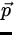= m(1)
Note:
• Momentum is a vector.
• The units of linear momentum are: kg m/s.

We can rewrite Newton's Second Law in terms of momentum: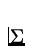= m= m=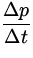. (2)
Interpretation: Force can be expressed as: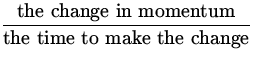.

Definition: Impulse (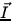)=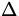t =. (3)
Note: Usually the force is a strongly dependent function of time. In this case, we need to use the average force in the above equation.